# Texas Go Math Grade 5 Lesson 4.5 Answer Key 2-Digit Divisors

Refer to our Texas Go Math Grade 5 Answer Key Pdf to score good marks in the exams. Test yourself by practicing the problems from Texas Go Math Grade 5 Lesson 4.5 Answer Key 2-Digit Divisors.

## Texas Go Math Grade 5 Lesson 4.5 Answer Key 2-Digit Divisors

Unlock the Problem

The annual rainfall in Greensville is 4.32 inches. What is the average monthly rainfall in Greensville?

One Way Use place value.
Divide. 4.32 ÷ 12

STEP 1: Share the ones.4 ones cannot be shared among 12 groups without regrouping.
Place a zero to show there are no ones.

STEP 2: Share the tenths.Divide. _______ tenths ÷ 12
Multiply. 12 × _______ tenths
Subtract. _______ tenths – _______ tenths
Check._______ tenths cannot be shared among 12 groups.

STEP 3: Share the hundredths.Divide. ______ hundredths ÷ 12
Multiply. 12 × _______ hundredths
Subtract, _______ hundredths – _______ hundredths
Check. _______ hundredths cannot be shared among 12 groups.
Place the decimal point in the quotient to separate the ones and the tenths.

So, the average monthly rainfall in Greenville is ___________ inch.

STEP 1: Share the ones.4 ones cannot be shared among 12 groups without regrouping.
Place a zero to show there are no ones.

STEP 2: Share the tenths.Divide. 43 tenths ÷ 12
Multiply. 12 × 3 tenths
Subtract. 43tenths – 36tenths
Check.7 tenths cannot be shared among 12 groups.

STEP 3: Share the hundredths.Divide. 72 hundredths ÷ 12
Multiply. 12 × 6 hundredths
Subtract, 72 hundredths – 72hundredths
Check. 0 hundredths cannot be shared among 12 groups.
Placed the decimal point in the quotient to separate the ones and the tenths.

So, the average monthly rainfall in Greenville is 0.36 inch.

Math Talk
Mathematical Processes

Explain how you would model 10.32 ÷ 12 using base-ten blocks.

Another Way Use an estimate.

Divide as you would with whole numbers.
Divide. $40.89 ÷ 47 • Estimate the quotient. 4,000 hundredths ÷ 50 = 80 hundredths, or$0.80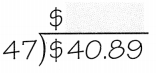• Divide the tenths.
• Divide the hundredths. When the remainder is zero and there are no more digits in the dividend, the division is complete.
• Use your estimate to place the decimal point. Place a zero to show there are no ones.

So, $40.89 ÷ 47 is$0.80.
Answer: The estimated quotient is 0.80
Explanation:Explain how you used the estimate to place the decimal point in the quotient.
Estimated to place the decimal point.
Placed a zero to show there are no ones.
the decimal point is placed in the product so that the number of decimal places in the product is the sum of the decimal places in the factors.

Share and Show

Divide.

Question 1.Estimate the quotient.
40 tenths ÷ 20 = _________Explanation:
Divided the tenths.
Divide the hundredths.
When the remainder is zero and there are no more digits in the dividend, the division is complete.
Used your estimate to place the decimal point. Place a zero to show there are no ones.

Question 2.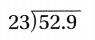Estimate the quotient.
60 tenths ÷ 20 =3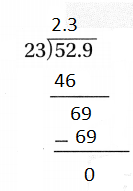Explanation:
Divided the tenths.
Divide the hundredths.
When the remainder is zero and there are no more digits in the dividend, the division is complete.
Used your estimate to place the decimal point. Place a zero to show there are no ones.

Question 3.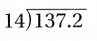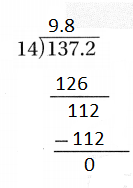Estimate the quotient.
140 tenths ÷ 14 = 10
Explanation:
Divided the tenths.
Divide the hundredths.
When the remainder is zero and there are no more digits in the dividend, the division is complete.
Used your estimate to place the decimal point. Place a zero to show there are no ones.

Question 4.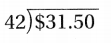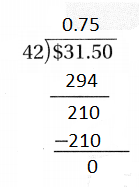Estimate the quotient.
40 tenths ÷ 40 = 1
Explanation:
Divided the tenths.
Divide the hundredths.
When the remainder is zero and there are no more digits in the dividend, the division is complete.
Used your estimate to place the decimal point. Place a zero to show there are no ones.

Problem Solving

Question 5.
H.O.T. Representations Make a model to find 11.16 ÷ 18. Describe your model.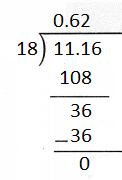2 digit division model
Explanation:
Divided the tenths.
Divide the hundredths.
When the remainder is zero and there are no more digits in the dividend, the division is complete.
Used your estimate to place the decimal point. Place a zero to show there are no ones.

Question 6.
Write Math what’s the Error? Darla divided 812.5 by 50. She says the quotient is 1.625. Describe Darla’s error.
Explanation:
She placed the decimal point wrong

Problem Solving

Question 7.
Jin makes trail mix with apricots and walnuts. A package of dried apricots weighs 25.5 ounces. Jin divides the apricots equally among 34 bags of trail mix. How many ounces of apricots are in each bag?
Explanation:
Jin makes trail mix with apricots and walnuts.
A package of dried apricots weighs 25.5 ounces.
Jin divides the apricots equally among 34 bags of trail mix.
2.5 ÷ 34 = 0.75

Question 8.
Analyze A large box of cereal weighs 17 ounces and costs $5.95. A small box of the same cereal weighs 10 ounces and costs$3.90. Which has a greater cost per ounce?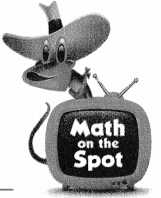Answer: A large box of cereal weighs 17 ounces and costs $5.95. is greater cost per ounce Explanation: A large box of cereal weighs 17 ounces and costs$5.95.
17 ÷ 5.95 = 2.85
A small box of the same cereal weighs 10 ounces and costs $3.90. 10 ÷ 3.90 = 2.56 2.85 – 2.56 = 0.29 Question 9. Multi-Step Maya trains 5 days each week for a triathlon. In 5 weeks she logs 24.6 miles in the pool. 445.45 miles on the bike, and 167.45 miles running. On average, how many miles did Maya cover each day? Answer: 318.5miles Explanation: Maya trains 5 days each week for a triathlon. In 5 weeks she logs 24.6 miles in the pool. 445.45 miles on the bike, and 167.45 miles running. 24.6 + 445.45 + 167.45 = 637.5 To find the average we have to divide the total with 2 637.5 ÷ 2 = 318.5 Daily Assessment Task Fill in the bubble completely to show your answer. Question 10. A scientist conducting a dig spent$37.95 on 23 packets of hand wipes for her team of volunteers. What was the price of each packet?
(A) $16.50 (B)$16.05
(C) $1.60 (D)$1.65
Explanation:
A scientist conducting a dig spent $37.95 on 23 packets of hand wipes for her team of volunteers. the price of each packet is$1.65

Question 11.
Calvin needs to buy carpet to cover the floor of a rectangular room with an area of 170.8 square feet. Calvin measures the room’s length to be 14 feet. He then divides the room’s area by its length to find the room’s width. How many decimal places will the quotient have?
(A) 0
(B) 1
(C) 2
(D) 3
Explanation:
Calvin needs to buy carpet to cover the floor of a rectangular room with an area of 170.8 square feet.
Calvin measures the room’s length to be 14 feet.
He then divides the room’s area by its length to find the room’s width.
The quotient is 12.2 so, the decimal point is 2

Question 12.
Multi-Step Farmer Lee grows tomatoes and squash. He harvests 49.92 kilograms of tomatoes and 65.92 kilograms of squash. He distributes the tomatoes and squash into 32 farm share baskets. How many more kilograms of squash than tomatoes does each basket contain?
(A) 2.06 kilograms
(B) 0.5 kilogram
(C) 1.56 kilograms
(D) 3.62 kilograms
Explanation:
Farmer Lee grows tomatoes and squash.
He harvests 49.92 kilograms of tomatoes and 65.92 kilograms of squash.
He distributes the tomatoes and squash into 32 farm share baskets.
49.92 ÷ 32 = 2.06
65.92 ÷ 32 = 1.56
2.06 – 1.56 = 0.5
0.5 more kilograms of squash than tomatoes in each basket contain.

Texas Test Prep

Question 13.
Jasmine uses 14.24 pounds of fruit for 16 servings of fruit salad. If each serving contains the same amount of fruit, how much fruit is in each serving?
(A) 0.089 pound
(B) 1.76 pounds
(C) 0.89 pound
(D) 17.6 pounds
Explanation:
Jasmine uses 14.24 pounds of fruit for 16 servings of fruit salad.
If each serving contains the same amount of fruit,
the fruit in each serving is 0.89 pounds

### Texas Go Math Grade 5 Lesson 4.5 Homework and Practice Answer Key

Divide.

Question 1.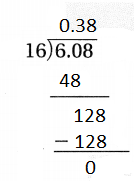Explanation:
Divided the tenths.
Divide the hundredths.
When the remainder is zero and there are no more digits in the dividend, the division is complete.
Used your estimate to place the decimal point. Place a zero to show there are no ones.

Question 2.Explanation:
Divided the tenths.
Divide the hundredths.
When the remainder is zero and there are no more digits in the dividend, the division is complete.
Used your estimate to place the decimal point. Place a zero to show there are no ones.

Question 3.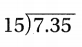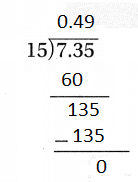Explanation:
Divided the tenths.
Divide the hundredths.
When the remainder is zero and there are no more digits in the dividend, the division is complete.
Used your estimate to place the decimal point. Place a zero to show there are no ones.

Question 4.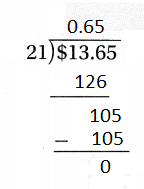Explanation:
Divided the tenths.
Divide the hundredths.
When the remainder is zero and there are no more digits in the dividend, the division is complete.
Used your estimate to place the decimal point. Place a zero to show there are no ones.

Question 5.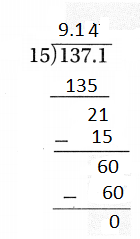Explanation:
Divided the tenths.
Divide the hundredths.
When the remainder is zero and there are no more digits in the dividend, the division is complete.
Used your estimate to place the decimal point. Place a zero to show there are no ones.

Question 6.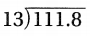Explanation:
Divided the tenths.
Divide the hundredths.
When the remainder is zero and there are no more digits in the dividend, the division is complete.
Used your estimate to place the decimal point. Place a zero to show there are no ones.

Question 7.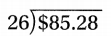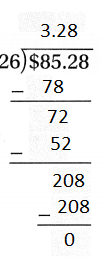Explanation:
Divided the tenths.
Divide the hundredths.
When the remainder is zero and there are no more digits in the dividend, the division is complete.
Used your estimate to place the decimal point. Place a zero to show there are no ones.

Question 8.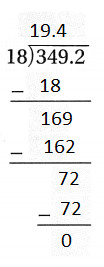Explanation:
Divided the tenths.
Divide the hundredths.
When the remainder is zero and there are no more digits in the dividend, the division is complete.
Used your estimate to place the decimal point. Place a zero to show there are no ones.

Question 9.Explanation:
Divided the tenths.
Divide the hundredths.
When the remainder is zero and there are no more digits in the dividend, the division is complete.
Used your estimate to place the decimal point. Place a zero to show there are no ones.

Question 10.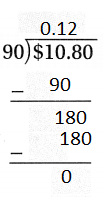Explanation:
Divided the tenths.
Divide the hundredths.
When the remainder is zero and there are no more digits in the dividend, the division is complete.
Used your estimate to place the decimal point. Place a zero to show there are no ones.

Question 11.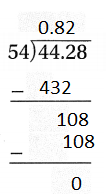Explanation:
Divided the tenths.
Divide the hundredths.
When the remainder is zero and there are no more digits in the dividend, the division is complete.
Used your estimate to place the decimal point. Place a zero to show there are no ones.

Question 12.Explanation:
Divided the tenths.
Divide the hundredths.
When the remainder is zero and there are no more digits in the dividend, the division is complete.
Used your estimate to place the decimal point. Place a zero to show there are no ones.

Problem Solving

Question 13.
Carla’s car travels 412.5 miles on a tank of gas. The tank holds 15 gallons of gas. How many miles can Carla go on each gallon?
Explanation:
Carla’s car travels 412.5 miles on a tank of gas.
The tank holds 15 gallons of gas.
Carla can go on each gallon of gas is 27.5 miles

Question 14.
Muffins cost $35.40 for a dozen or$18.72 for a half dozen. Which is the better buy? Explain.
Answer: Dozen = $2.95 Half dozen =$3.12
Explanation:
The better buy is dozen as it cost per piece is $2.95 Lesson Check Fill in the bubble completely to show your answer. Question 15. Anita pays$20.70 to copy an 18 page report. What is the cost for each page?
(A) $1.05 (B)$1.03
(C) $1.15 (D)$1.10
Explanation:
Anita pays $20.70 to copy an 18 page report. the cost for each page is$1.15

Question 16.
A florist sells a dozen roses for $29.88. What is the cost of one rose? (A)$2.41
(B) $2.49 (C)$2.40
(D) $2.08 Answer: B Explanation: A florist sells a dozen roses for$29.88.
cost of one rose $2.49 since dozen equals to 12. So 29.88 ÷ 12 = 2.49 Question 17. Cameron has a stack of 13 identical books that is 30.55 centimeters tall. He divides the total height by the number of books to find the width of one book. How many decimal places will the quotient have? (A) 3 (B) 2 (C) 1 (D) 0 Answer: Explanation: Cameron has a stack of 13 identical books that is 30.55 centimeters tall. He divides the total height by the number of books to find the width of one book. So width of each book is 2.35cm. Since 30.55 ÷13 = 2.35. So 2 decimal places will the quotient have. Question 18. Kiera makes 188.6 ounces of punch for a pool party. She has 23 guests attending the party. How many ounces of punch does she make for each guest? (A) 8.2 ounces (B) 9.4 ounces (C) 8.1 ounces (D) 7.2 ounces Answer: A Explanation: Kiera makes 188.6 ounces of punch for a pool party. She has 23 guests attending the party. So She made the 8.2 ounces of punch for each guest. Since 188.6 ÷ 23 = 8.2 Question 19. Multi-Step Last year, Mr. Henderson paid a total of$98.40 for phone service and $79.20 for garbage pickup. What was his average cost per month for phone service and garbage pickup? (A)$8.20
(B) $6.60 (C)$1.48
(D) $14.80 Answer: D Explanation: Last year, Mr. Henderson paid a total of$98.40 for phone service and $79.20 for garbage pickup. So total paid per year is$177.6.
12 months in a year. So his average cost per month phone service and garbage pick up is 14.80.
Since 177.60 ÷12 = 14.80

Question 20.
Multi-Step Isabel worked 20 hours last week and earned $145.80. Nan worked 15 hours last week and earned$112.50. How much more does Nan earn per hour?
(A) $2.22 (B)$3.30
(C) $0.39 (D)$0.21
Isabel worked 20 hours last week and earned $145.80. So Isabel earn$7.29 per hour. Since 145.80 ÷20 = 7.29.
Nan worked 15 hours last week and earned $112.50. So Nan earn$7.50 per hour. Since $112.50 ÷15 = 7.50. Nan Earned$0.21 more than Isabel per hour.